Revision Notes: Evaluation

# Revision Notes: Evaluation Notes | Study Artificial Intelligence for Class 10 - Class 10

## Document Description: Revision Notes: Evaluation for Class 10 2022 is part of Evaluation for Artificial Intelligence for Class 10 preparation. The notes and questions for Revision Notes: Evaluation have been prepared according to the Class 10 exam syllabus. Information about Revision Notes: Evaluation covers topics like Introduction to Evaluation Artificial Intelligence and Revision Notes: Evaluation Example, for Class 10 2022 Exam. Find important definitions, questions, notes, meanings, examples, exercises and tests below for Revision Notes: Evaluation.

Introduction of Revision Notes: Evaluation in English is available as part of our Artificial Intelligence for Class 10 for Class 10 & Revision Notes: Evaluation in Hindi for Artificial Intelligence for Class 10 course. Download more important topics related with Evaluation, notes, lectures and mock test series for Class 10 Exam by signing up for free. Class 10: Revision Notes: Evaluation Notes | Study Artificial Intelligence for Class 10 - Class 10
 1 Crore+ students have signed up on EduRev. Have you?

Introduction to Evaluation Artificial Intelligence

The last stage of AI Project cycle is evaluation. When the model or project is ready after problem scoping, data acquisition, data exploration and modelling, the final stage is to just evaluate the model or project whether it is ready or not for the action.

Evaluation helps to check whether the model is better than the other one or not. It is the most important part of the development process. It helps to determine the best model for data processing. It also helps in how well the model will work in future.

What is evaluation ?

As we know we have two kinds of datasets:

• Training Data Set
• Testing Data Set
• Evaluation is the process of understanding the reliability of any AI model, based on outputs by feeding the test dataset into the model and comparing it with actual answers.
• There can be different Evaluation techniques, depending of the type and purpose of the model.
• It’s not recommended to use the data we used to build the model to evaluate it. This is because our model will simply remember the whole training set, and will therefore always predict the correct label for any point in the training set. This is known as overfitting.

Consider this scenario where you have an AI prediction model which predicts the possibilities of fires in the forest. The main aim of this model is to predict whether a forest fire has broken out into the forest or not. To understand whether the model is working properly or not we need to predict to check if the predictions made by the model is correct or not.

So there are two conditions:

• Prediction
• Reality

Prediction

The output given by the machine after training and testing the data is known as a prediction.

Reality

The reality is the real situation or real scenario in the forest where the prediction has been made by a machine.

There are four cases and all have their own terms: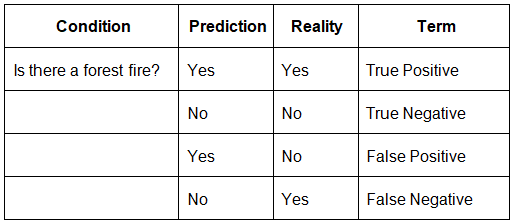Condition 1 – Prediction – Yes, Reality – Yes (True Positive)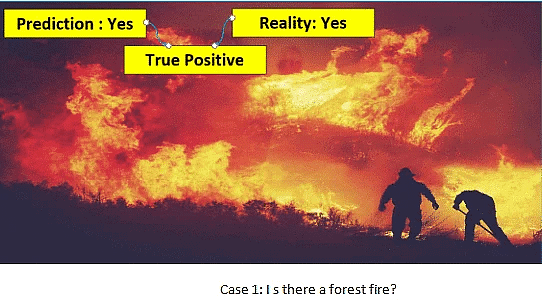This condition arises when the prediction and reality both match with yes (prediction – yes, reality – yes), if forest fire has broken out.

Condition 2 – Prediction – No, Reality – No (True Negative)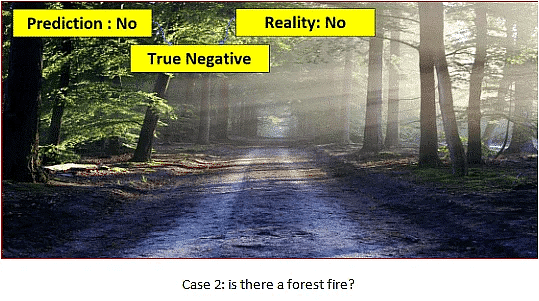If there is no fire in the forest and prediction predicted by machine correctly as No as well as reality also no, this condition is known as True Negative.

Condition 3 – Prediction – Yes, Reality – No (False Positive)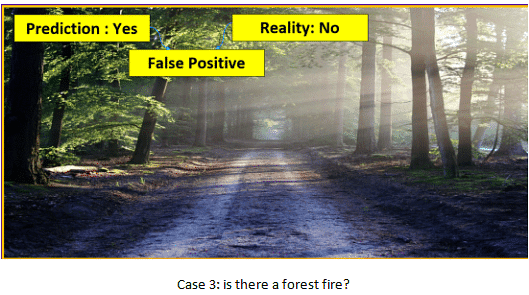There is no fire in reality but the machine has predicted yes incorrectly. This condition is known as False Positive.

Condition 4 – Prediction – No, Reality – Yes (False Negative)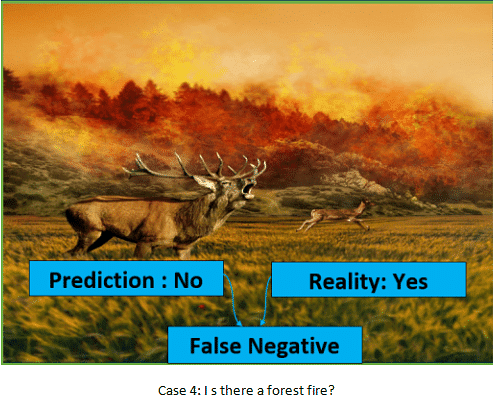The forest fire has broken out in reality but the machine has incorrectly predicted No refers to False Negative condition.

Confusion Matrix

It is a comparison between prediction and reality. It helps us to understand the prediction result. It is not an evaluation metric but a record that can help in evaluation. Observe the four conditions explained above.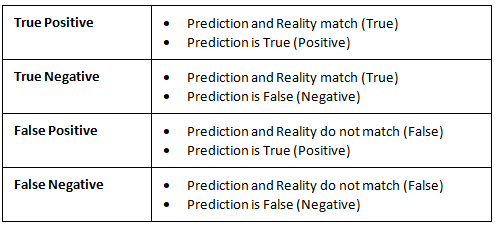Prediction and reality can be mapped together with the help of a confusion matrix. Look at the following: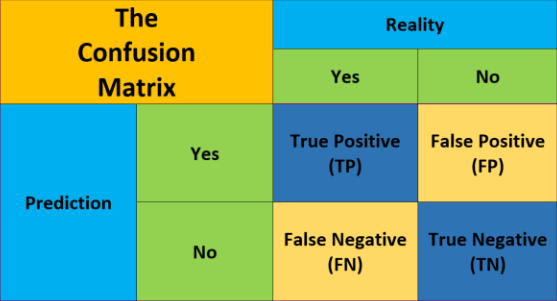Evaluation Methods

These evaluation methods are as follows:

• Accuracy
• Precision
• Recall
• F1 Score

1. Accuracy

• The percentage of correct predictions out of all the observations is called accuracy.
• If the prediction matches with reality then it said to be correct.
• There are two conditions where prediction matched with reality:
• True Positive
• True Negative
• So the formula for accuracy is: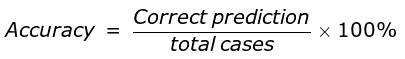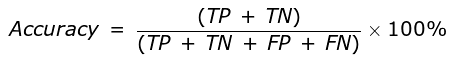The total observations cover all the possible cases of prediction that can be True Positive (TP), True Negative (TN), False Positive (FP), and False Negative (FN).

Accuracy talks about how true the predictions are by any model. Now let’s return to the forest fire example.

If the model always predicts that there is no fire where in reality there is a 2% chance of forest fire breaking out. So 98% of the model is right but for these 2% also model predicts there is no fire. Hence the elements of the formula are as follows:

• True Positive = 0
• True Negatives = 98
• Total Cases = 100

Therefore, accuracy = (98 + 0) / 100 = 98%.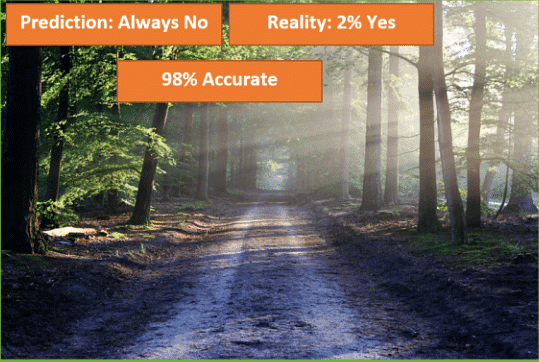This returns high accuracy for an AI model. But the actual cases where the fire broke out are not taken into account. Therefore there is a need to look at another parameter that takes account of such cases as well.

Precision

• The percentage of true positive cases versus all the cases where the prediction is true.
• It takes into account the True Positives and False Positives.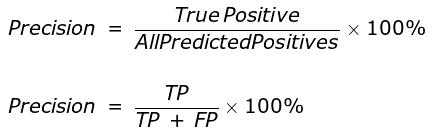Now return to the forest fire example, Assume that model predictions of forest fire is irrespective of the reality. In this case, all the positive conditions will be taken into the account i.e. True Positive (TP) and False Positive (FP). So in this case firefighters always see if the alarm was True or False.

Recall the story of the boy who falsely cries out and complains about the wolf every time, but when the wolf came in reality, no one rescued him. Similarly if Precision is low (more false alarms) then the firefighters would get complacent and might not go and check every time considering it a false alarm.

So, if Precision is high, means that True Positive cases are more, given lesser False alarms.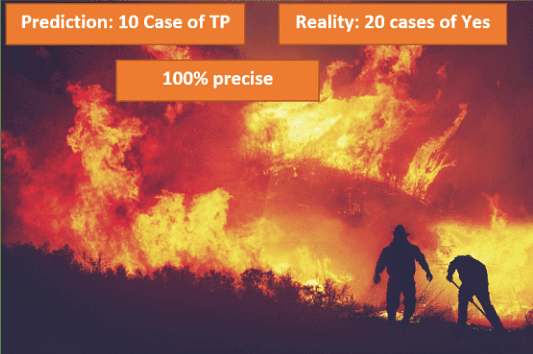• Now consider that a model has 100% precision. It means the machine says there’s a fire, which is considered as True Positive.
• In the same model, there can be a rare exceptional case where there was actual fire but the system could not detect it in false negtive case.
• But till the precision value would not be affected by it because it does not take FN into account.
• Now think Is precision then a good parameter for model performance?

Recall

In the recall method, the fraction of positive cases that are correctly identified will be taken into consideration. It majorly takes into account the true reality cases wherein Reality there was a fire but the machine either detected it correctly or it didn’t. That is, it considers True Positives and False Negatives.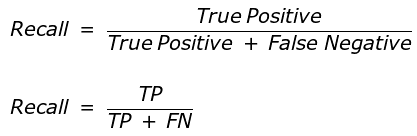As we have observed that the numerator in both precision and recall is same i.e. True Positive. Where in the denominator, precision counts the False Positive while recall considers False Negatives. In the following case False negative cases can be very costly.

• In forest fire case false negative cost us a lot and are risky too. If no alarm is given when there is a forest fire, maybe the whole forest burn down.
• Viral Outbreak is also one of False Negative case. Consider the case of covid 19, as it spreads but the machine did not detect it then imagine it can affect so many lives.

Sometimes False Positive also costs us more than False Negative. Just have a look at the following cases:

• Mining imagine a model tell us about the existence of treasure at a point and keep on digging there but it turns out that it is false alarm. Here False Positive case can be very costly.
• Spam e-mails, If the model always predicts that the email is spam, people may not look at it and finally might lose important information.

You can think of more examples of these cases. Observe this table: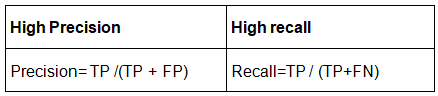So if you want to know the performance of your model is good, you need these two measures:

• Recall
• Precision

In some cases, high precision may be there but low recall and for some cases, low precision may be there but high recall. Hence both measures are very important. So there is a need for a parameter that takes both Precision and Recall into account.

F1 Score

F1 score is the balance between precision and recall.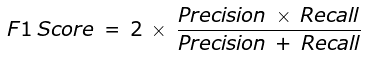• When we have 1 value (100%) for both Precision and Recall which is ideal solution.
• In that case the F1 score would be also ideal 1 (100%).
• It is known as the perfect value for F1 score.
• As the values of both Precision and Recall ranges from 0 to 1, the F1 score also ranges from 0 to 1.
• Now let us explore the variations we have in F1 score: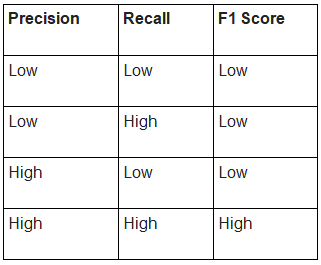In conclusion, we can say that a model has good performance if the F1 Score for that model is high.

The document Revision Notes: Evaluation Notes | Study Artificial Intelligence for Class 10 - Class 10 is a part of the Class 10 Course Artificial Intelligence for Class 10.
All you need of Class 10 at this link: Class 10

## Artificial Intelligence for Class 10

13 videos|14 docs|4 tests
 Use Code STAYHOME200 and get INR 200 additional OFF

## Artificial Intelligence for Class 10

13 videos|14 docs|4 tests

Track your progress, build streaks, highlight & save important lessons and more!

,

,

,

,

,

,

,

,

,

,

,

,

,

,

,

,

,

,

,

,

,

;